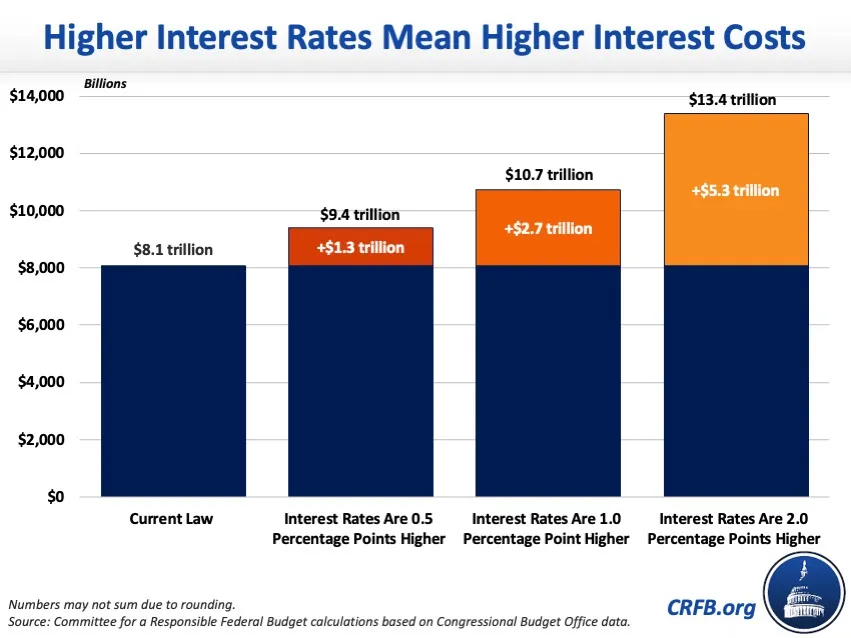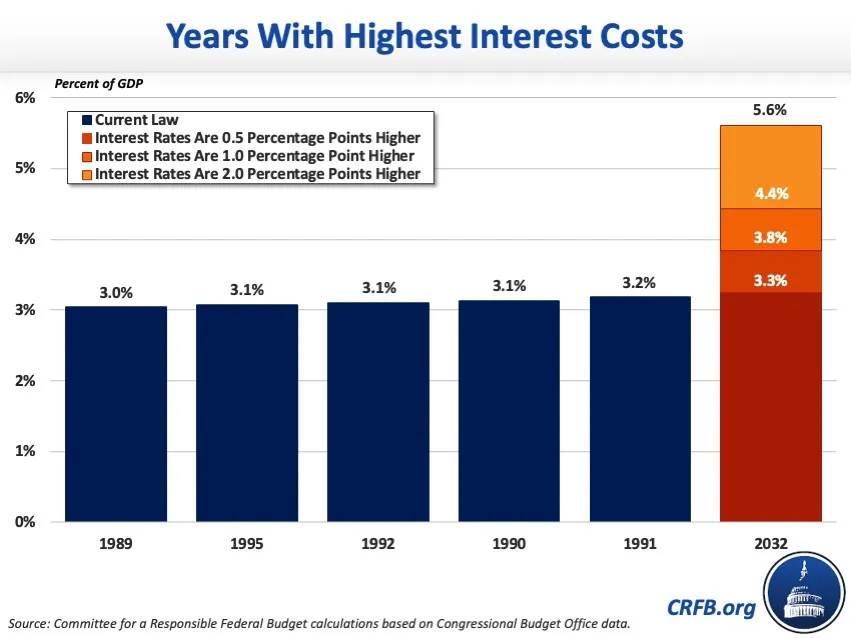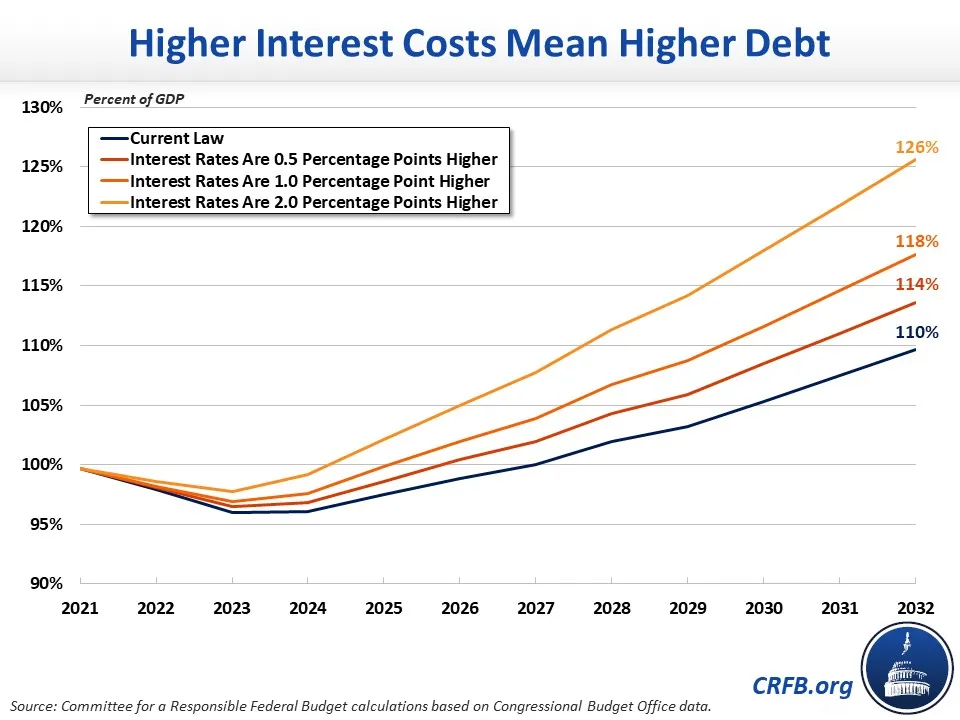# What Would Higher Interest Rates Mean for the Debt?

Interest payments on the national debt are projected to be the fastest-growing part of the federal budget. Under the Congressional Budget Office's (CBO) latest baseline, interest costs will triple from nearly \$400 billion in Fiscal Year (FY) 2022 to \$1.2 trillion in 2032, and total \$8.1 trillion over the next decade. As a share of the economy, net interest will reach a record 3.3 percent of Gross Domestic Product (GDP) by 2032, surpassing the prior record of 3.2 percent of GDP set in 1991. In reality, however, interest rates - and in turn interest costs - could be even higher.

CBO's latest economic forecast projects the interest rate on three-month Treasury notes will average 0.9 percent in calendar year 2022 and then rise to 2.6 percent by 2025 before falling to 2.3 percent by 2032, while the interest rate on ten-year Treasury notes will average 2.4 percent in 2022 and rise to 3.8 percent by 2032. In reality, however, current interest rates are well above CBO's forecast and there is growing pressure on the Federal Reserve to raise short-term rates even higher than previously assumed.

In this analysis, we show that:

• If interest rates are 50 basis points (0.5 percentage points) above CBO's forecast, interest payments would total \$9.4 trillion over the FY 2023 to 2032 period and budget deficits would increase by an average of \$143 billion per year.
• If interest rates are 100 basis points (one percentage point) above CBO's forecast, interest payments will total \$10.7 trillion over the next decade and budget deficits will increase by an average of \$285 billion per year.
• If interest rates are 200 basis points (two percentage points) above CBO's forecast, interest payments will total \$13.4 trillion over the next decade and budget deficits would increase by an average of \$570 billion per year
• These scenarios would boost debt-to-GDP in FY 2032 by 4 to 16 percentage points, to as high as 126 percent of GDP and well above the 110 percent of GDP projected under current law. Interest costs in 2032 could reach as high as 5.6 percent of GDP, well above the current law projection of 3.3 percent and the prior record of 3.2 percent of GDP.

### Higher Interest Rates Would Increase Net Interest Costs

At just over 3.4 percent, the average interest rate on ten-year Treasury notes is about 90 basis points (0.9 percentage points) above the 2.5 percent CBO projected it would be this quarter. While interest rate projections are highly uncertain, if they continue to outperform CBO's forecast the result will be more debt and more spending needed to service that debt.Under current law, CBO estimates that net interest spending will total \$8.1 trillion (2.6 percent of GDP) between FY 2023 and 2032, or an average of \$808 billion per year. If interest rates on the projected annual debt stock are 50 basis points (0.5 percentage points higher) than what CBO currently projects - reflecting a continuation of the current disparity between reality and forecasts - interest costs would increase by an average of \$133 billion per year and interest costs would total \$9.4 trillion (3.0 percent of GDP) trillion through 2032, a \$1.3 trillion (0.4 percent of GDP) increase from current law. Interest costs in 2032 alone would total 3.8 percent of GDP.If interest rates are 100 basis points (one percentage point) above CBO's forecast, interest costs would be an average of \$265 billion higher per year and total \$10.7 trillion (3.4 percent of GDP) over the next decade, a \$2.7 trillion (0.8 percent of GDP increase from current law. Net interest in FY 2032 alone would be 4.4 percent of GDP.  Should interest rates increase further and end up being 200 basis points (two percentage points) above CBO's forecast, average annual interest payments would be \$531 billion higher. Interest costs through FY 2032 would total \$13.4 trillion (4.3 percent of GDP), which would be a \$5.3 trillion (1.7 percent of GDP) increase from current law. Under this scenario, net interest would total 5.6 percent of GDP in 2032.

### Higher Interest Rates Would Increase Deficits and Debt

Higher interest costs would increase annual budget deficits and the national debt. CBO projects that under current law budget deficits will total \$15.7 trillion over the FY 2023-2032 period. After including the revenue effects and higher interest costs that would stem from higher interest rates, budget deficits would be \$1.4 trillion larger (\$17.2 trillion total deficits) than under current law if interest rates are 0.5 percentage points higher. With interest rates that are one percentage point higher, deficits would be \$2.9 trillion larger (\$18.6 trillion total deficits). Interest rates that were two percentage points higher would increase deficits by \$5.7 trillion (\$21.4 trillion total deficits).

Under current law, debt is projected to reach a record 110 percent of GDP by FY 2032. If interest rates were 50 basis points higher, debt would reach 114 percent of GDP by 2032. Rates that were 100 or 200 basis points higher would push debt-to-GDP up to 118 and 126 percent, respectively, by 2032.As the national debt continues to rise and the interest rates on Treasury bills continue their upward trajectory, the federal government's interest payments on its debt are projected to grow rapidly through FY 2032. As a result, net interest will comprise a larger and larger share of federal spending, increasing deficits and crowding out other important priorities. Policymakers should work to reduce budget deficits and put debt on a sustainable path through a combination of entitlement reforms, spending reductions, and revenue increases.